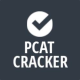# PCAT Breakdown: Quantitative Ability!

This is finally the final part aka part 7 of our series breaking down the Pharmacy Admission Test. Check out the complete series for our lowdown on the PCAT!

For our last trick we’re breaking down the Quantitative Ability section,

which coincidentally is also the last section you will face come test day!

You will have 45 minutes to answer 45 questions.

### Basic Math, 15%

Functions, Percentages, & Decimals

Unit Conversions

Log Base 10

### Algebra, 20%

Expressions, Equations, & Inequalities (e.g. solving problems using linear equations &                                                         equalities, solving quadratic equations, solving equations with radicals, etc.)

Functions (e.g. determining the inverses of functions, using max & min points, etc.)

### Statistics and Probability, 20%

Measures of Central Tendency

Variation

Graphical

Probability

Statistical Concepts

### Pre-Calculus, 22%

Functions (e.g. graphing and identifying domains, ranges, and intercepts, logarithms, graphing                                          inverses of circular functions, etc.)

Complex Numbers

Vectors (e.g. adding vectors graphically and algebraically, perform scalar multiplications, etc.)

### Calculus, 22%

Limits (e.g. finding limits of functions, infinite limits, etc.)

Continuity (e.g. interpret graphs of continuous and discontinuous functions)

Derivatives (e.g. finding derivatives by means of the sum & product, power rule, applying the                                   Mean Value Theorem, using implicit differentiation, etc.)

Integrals (e.g. finding antiderivatives and interpreting C, approximating areas bounded by                                                   curves, etc.)

Integration

#### Here’s a sample derivative question to give you an idea of what to expect…So there you have it Pre-Pharmers, our finally complete breakdown series on all things PCAT! Check out the blog for the rest of PCAT Cracker‘s section breakdowns and stay tuned for more things Pre-Pharm!

#### P.S. PRACTICE!